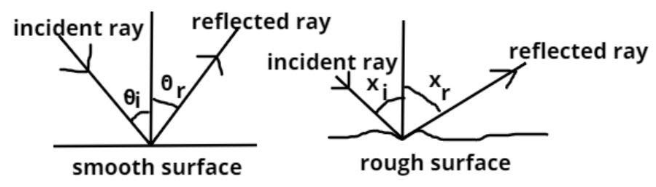# Angle of incidence is equal to the angle of reflection. This is true:(a) always(b) sometimes(c) under special condition(d) neverVerified
127.6k+ views
Hint – In this question think of the condition in which the light is incident upon a surface that is not smooth or rough, the angle of incidence is equal to the angle of reflection when we are dealing with smooth surfaces. But is it really true for a rough surface even is the main approach to this problem.

Complete step-by-step solution -As we know according to the law of reflection angle of incidence is equal to angle of reflection this is true if and only if, if the surface on which light strikes is smooth.
As shown in figure (1)
$\Rightarrow {\theta _i} = {\theta _r}$, where ${\theta _i}$ is angle of incidence and ${\theta _r}$ is angle of reflection.
If the surface is not smooth (i.e. if the surface has some irregularities) then the angle of incidence does not equal the angle of reflection.
As shown in figure (2)
$\Rightarrow {x_i} \ne {x_r}$, where ${x_i}$ is angle of incidence and ${x_r}$ is angle of reflection.
So angle of incidence is equal to angle of reflection this is true under special conditions.
So this is the required answer.
Hence option (C) is the correct answer.

Note – The laws of reflection are the fundamental principle behind the solution to this question, it states that when a ray of light reflects a smooth surface, the angle of incidence is always equal to the angle of reflection, and another law behind this rule is that at the point of incidence, the incident ray, the reflected ray and the normal ray all lie in the same plane.